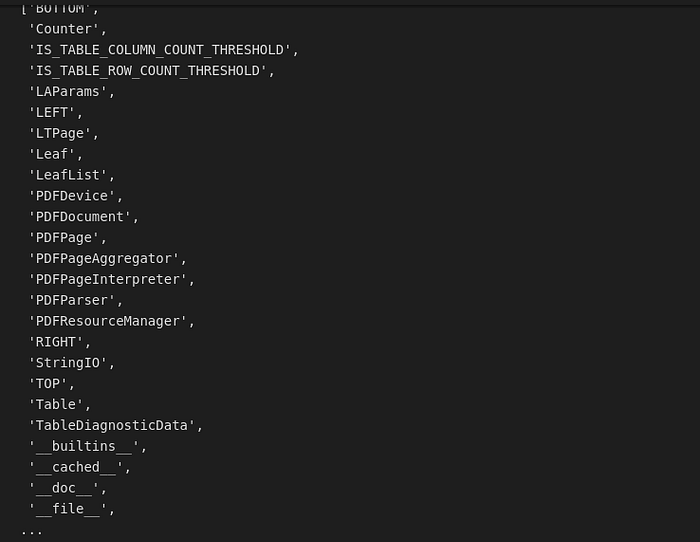## Practical Data Science: Extracting Tables from a PDF

• 2 months ago

When working with research papers, we sometimes would need to extract tables from the research papers to work with. There are several ways we can go about extracting these tables but we basically, will be talking about the more easier, accurate and stress free way.

Furthermore, after extracting these tables we may probably need them in excel format or in CSV or any preferred data format we may need them.

To begin, we need to get our development environment ready! I will be working with Jupyter notebook in Vs code. Let’s get started!

The first step would be to install a python library, using pip install (I would also install pdftables library). This will be the only python package to install if we have been working with Jupyter notebook before now, if not you will also want to pip install pandas our data frame work.

!pip install pdftables.six

This command line in our environment would install the pdftables and gets our package running. Now let’s import it using the next line of code.

import pdftables

Let us check out some cool commands we can use this library for, by running the next line of code.

dir(pdftables)We should get an output like this.

Next we will want to get the link to our pdf. It could either be a web link or a locally downloaded pdf.

file ="my_file_name.pdf"

Now I want to get the page number where my table is, so for mine it will only be a page number, so yours could be more than one. Another package(tabulate) enables us select all the pages at once and get all the tables, but most times its not so effective, probably how the pdf came, and there will be many fixing after extraction.

pg = pdftables.get_pdf_page(open(file, "rb"),13)# 13 there is specifying the page number

Lets see our table result

table= pdftables.page_to_tables(pg)table

Next, we would use the index method to get the row where our table columns can be found.

title = tabletitle

We will want to list out all the columns we need from the data.

allrows=[]for row_data in table[0:]:
allrows.extend([row_data[:4],row_data[4:]])allrows
#the number 4 there tells me there are 4 columns

The next step would be to import pandas and convert to a data frame.

import pandas as pddf=pd.DataFrame(allrows, columns=["1st column","2nd column", "3rd column", "4th column"])df

## Another python library for this

We can also make use of the camelot library. Let us see a quick walk through as well.

In our Jupyter notebook we can do the following installation.

! pip install 'camelot-py[cv]'

With the following two lines of code we can get our extracted tables from the whole pdf

import camelot as cam
pdd= cam.read_pdf("file", pages = 'all', flavor='stream')# you can choose a particular page number or use 'all'
# there are different flavour to use
# I prefer to use the stream

We can view our data frame by running the “pdd”

Conclusion

For this exercise we just concluded, we can convert our results to single or multiple excel sheets in an excel file. We would use the line of code below to achieve this.

with pd.ExcelWriter('output2.xlsx') as writer:
df.to_excel(writer, sheet_name='Sheet1')

In converting to csv we can use the line of code

df.to_csv (r'sheet.csv', index=None)

Thank you!!